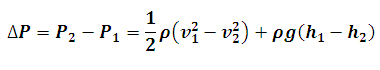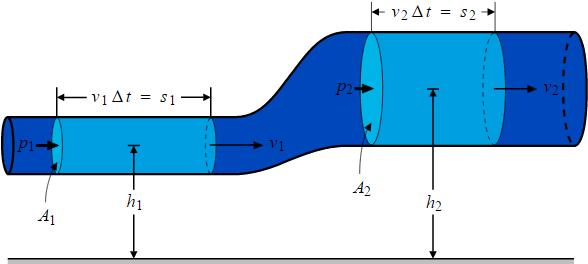# Bernoulli's Equation Calculator

This Calctown Calculator calculates the pressure difference between two points using bernoulli's equation.

m/s
m/s
m
m
kg/m3

#### Result

N/m2